If you have a related question, please click the "Ask a related question" button in the top right corner. The newly created question will be automatically linked to this question.

# OPA828: Setting time for OPA828

Part Number: OPA828

I find the below two settling time waveform in datasheet of INA828. What does the 12bits and 14 bits refer to? Why the settling time is different between 12bits and 14bits? Thanks.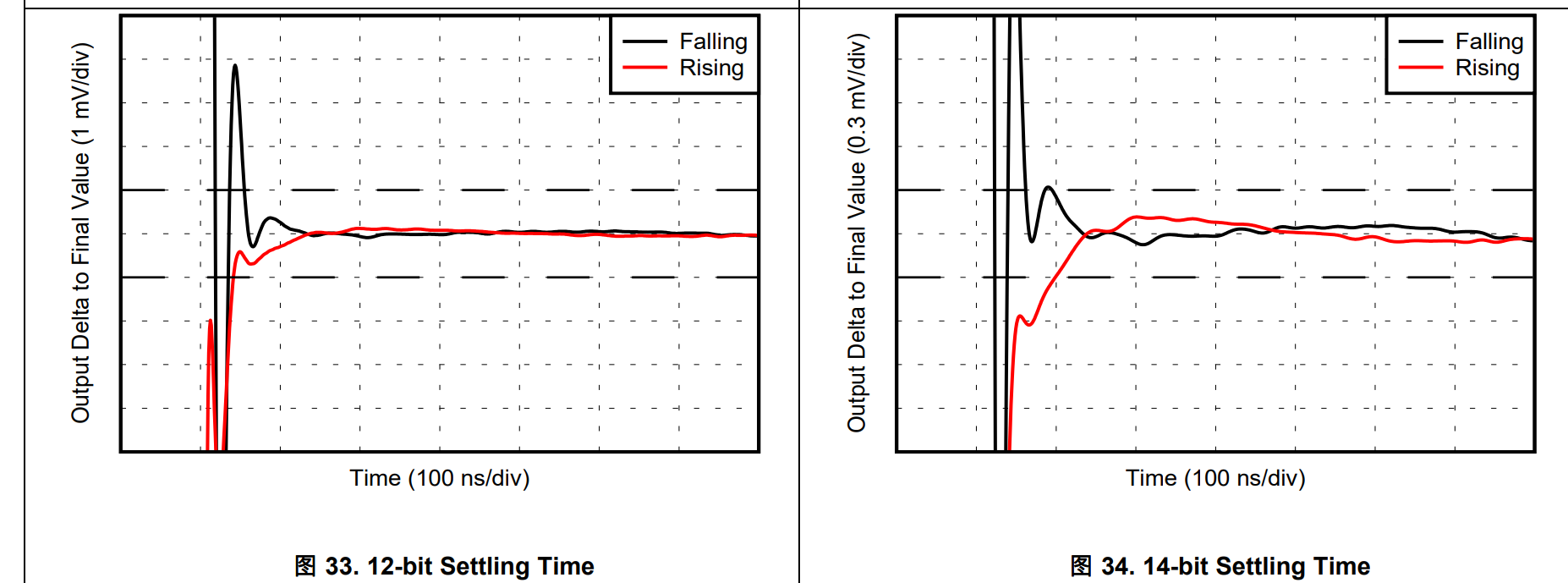• Hi Tess,

maybe it has to do with the circuit in section 8.2.1 of datasheet? Section 8.2.1.2 shows the situation for 16bit precision and a 0V to 5V swing. From this you can calculate the situation for 12bit and 14bit precision.

Kai

• Hi Kai,

Thanks for your reply. I notice that the acquisition time for ADS8860 is 290ns which I can find also in datasheet of ADS8860. But the settling time we talk about before is for OPA828 which is 120ns for 14bit and 110ns for 12bit.  I still have no idea how to calculate the situation for 12bit and 14bit precision?  And  what will effect the parameter of settling time for an apm?Please find 8.2.1.2 in the screenshot below. Thanks.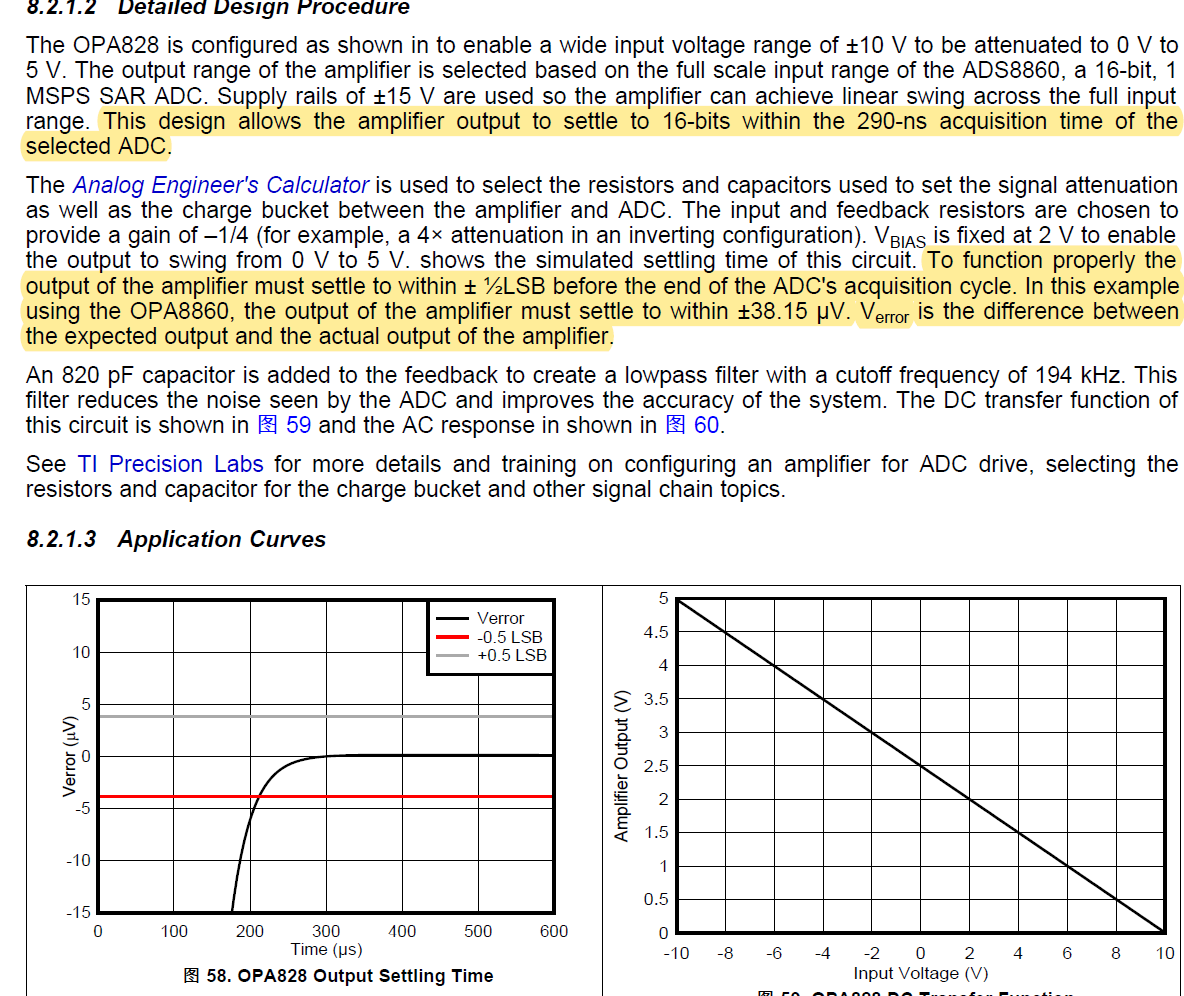Tess

• Hi Tess,

The settling time specification conditions are mentioned in the Electrical Characteristics Table on page 6 of the datasheet; these conditions correspond also to Figures 33 and 34.   In this case, the amplifier settling time is specified after a 10-V output step settling within ±0.0244% (12-bit resolution) or ±0.0061% (14-bit resolution) of the step size, when using a load capacitance of 30pF with the amplifier configured on the inverting configuration (G=-1).  In other words, this refers to settling within an error margin of ± (Step-Size)/ 2N , where N corresponds to the bit resolution, N=12 bit or N=14 bit .

Please note, the amplifier settling is dependent on the amplifier gain/circuit configuration, step size and output load conditions.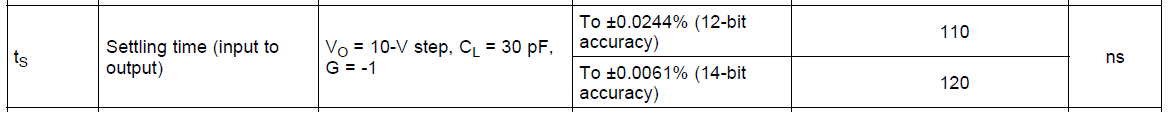Therefore, this settling time will be different when driving a SAR ADC such as the ADS8860. When driving a SAR ADC, the internal ADC sample-and-hold capacitor settling will depend on the external RC filter used between the amplifier and ADC, as well as the ADC’s internal sampling capacitor value and switch resistance, the ADC acquisition time, the ADC bit resolution and the ADC full-scale range.

The SAR ADC Least Significant Bit (LSB) resolution is typically calculated as LSB = (ADC-full-scale range) /2N  where N is the SAR ADC bit resolution.

If you wish to learn about amplifiers driving SAR ADCs, the precision lab sections below discuss in great detail how to select the amplifier and external RC filter, and simulate using the sample-and-hold settling using SPICE, given the SAR ADC full-scale range, sample-and-hold capacitor, switch resistance and acquisition time. There also many examples on the cookbook circuits of amplifiers driving ADCs that you may refer to.  Please see links below.

Best Regards,

Luis

These videos describe how to design the input driver circuitry for a successive approximation register analog-to-digital converter (SAR ADC).

ADC / Data Converter Circuit Cookbooks

Circuit examples include the design procedure, with calculations and SPICE simulations

http://www.ti.com/analog-circuit/circuit-cookbook.html

• You are right to be perplexed, that the little piece of the OPA828 data sheet you are referring to is full of errors. Here is Figure 57,

1. The input should be +/-10V, not just -10V

2. The DC bias on the V+ input is +2V, and should have a noise reduction cap across R4.

3. That 2V bias  at V+ gets a gain of 1.25 to set the output at 2.5V  with zero volt input signal, then the +/-10V produces the 0 to 5V swing reported.

4. The settling time in the spec tables is for one set of conditions, more importantly in this example the bandwidth is set to single pole by the feedback Cap to 194kHz. The will give a time constant for a step response of 820nsec.

5. For 1/2 LSB settling on a 16bit ADC, that is 5V/(2^(n+1) or the 38uV mentioned in the text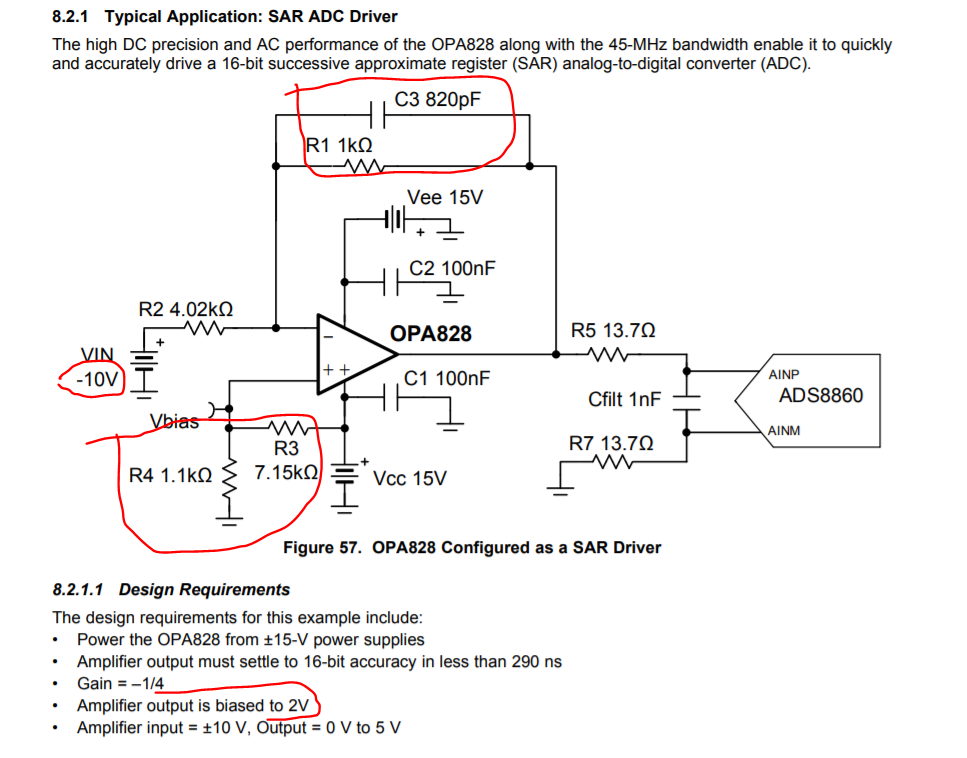In the next section, a number of errors also,

1. That OPA8860 should be the ADS8860. The response scale is highlighting a final window of +/-5uV not the 38uV mentioned earlier.

2. the simulated single pole settling shown cannot be for the bandlimited figure 57. not sure why it is so slow. The post RC pole is at 5.9MHz, not an issue.

3. The mapping from single pole bandwidth to settling within some final value is done by # of time constants. The time constant for the 200kHz Figure 57 is 796nsec, so it cannot be what was used to produce figure 58.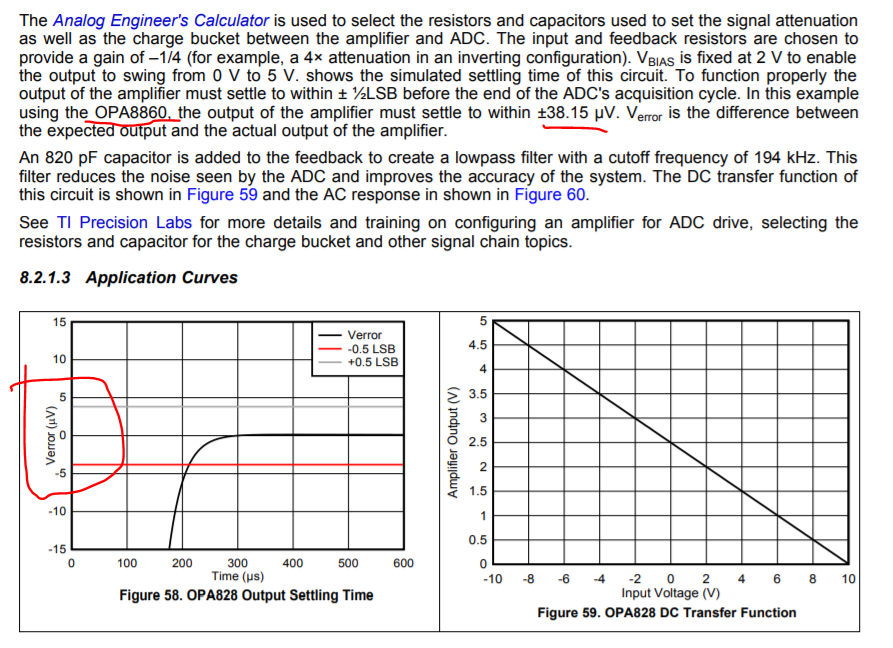Here is the ideal equation where n is the number of bits and tau is the time constant. This is for 1/2 LSB with that n+1 term, if you want 1/4 LSB make that an n+2. So in theory, for a 16bit adc, we need 11.8 time constants, which for the actual 800nsec with the feedback capacitor in Figure 7 would be 9.4usec. I must be missing something here.

Let us try to solve backwards on Figure 58 for accuracy, that 5uV for a 5V step works out to 20bits, Putting 2^(20) in to this equation, 14 time constants - no, can't make sense of this.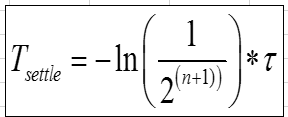• More possibilities -

1. 1st of all the x-axis of Figure 58 probably should be nsec.

2. With no feedback C, Figure 57 has a BW set by the post RC filter at 5.8Mhz or a 27.4nsec time constant,

3. then the 200nsec in Figure 58 would be 7.3time constants - no not enough for that level of accuracy -

This section 8.2.1 tis a mystery,

And, going back to the spec tables - these are fraught with hazard as well - with shifting conditions, So the gain of -1 is used for settling time. Figure 23 shows about 35MHz SSBW with some peaking. You never know if they slowed the input edge down to stay out of slew limiting but the approximate peak dV/dT for a 35MHz 2nd order reponse is 2.85*10V*35MHz = 997V/usec if they did not slow the input edge down. I would be guessing they did not.

So a 12bit settling is 9 time constants or 9*4.5nsec = 40nsec. So the 110nsec shown might include some slewing time. 10V/150V/usec = 67nsec. so yes, I would guess this a slewing step, then using another 40nsec of recovering to linear settling time.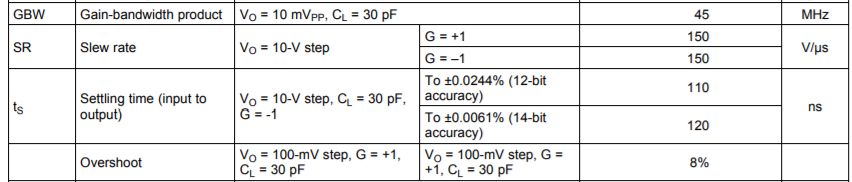• There was another similar discussion recently here - for the higher speed parts, I would typically set a test input edge rate to stay out of slew limiting to get better settling time. I was surprised to find a recent ADI part did not - just a judgement call, we tend to forget in the lab that we have faster sources the most systems can produce or would need to.

• HI Steffes,

You are correct that the circuit on section 8.2.1.2  is not related to the input-output settling specifications on the electrical table.

I believe the author of the circuit on section 8.2.1.2 is discussing the amplifier's output settling to 1/2-LSB error of the ADS8860 after simulating with SPICE the SAR ADC sample-and-hold charge/discharge behavior during the acquisition and conversion process.

The key difference, in the ADC circuit,  the transient step only occurs at the OPA828 output.  In this OPA828+ADS8860 circuit, the small transient step is at the amplifier output, as the internal 60-pF sample-and-hold capacitor partially discharges on every conversion.  This transient step at the OPA828 is relatively small, since the 1-nF capacitor (external Cfilt capacitor above) is much larger than the internal ~60-pF sample-and-hold that is getting charged and discharged during the SAR's acquisition and conversion process.

I believe the note "Input =+/-10V" indicates the input voltage range permissible in the circuit, but I don't believe is indicating an actual input step in this circuit (I will ask the author to clarify).

There is definitely  error on the x-axis of figure 58, this should be in the nano-seconds range, which would correlate to the SAR ADC acquisition time (the text also mentions settling to 16-bit accuracy in 290-ns). Using the ADS8860 with VREF=5V,  the 16-Bit ADC Least Significant bit is 5-V / 2^18 = 76uV; therefore 1/2 LSB = 38uV.

I will provide the feedback to the author, in order to clarify both the settling spec of the OPA828, and clarify all the conditions of the application circuit on 8.2.1.2; which are not related.  I believe the electrical table discusses the amplifiers input-output settling after an input step signal; and the other is discussing the settling of a SAR ADC's internal sample-and-hold capacitor, where some charge loss occurs in the SAR ADC's internal 60-pF sample-and-hold capacitor.

Best Regards,

Luis